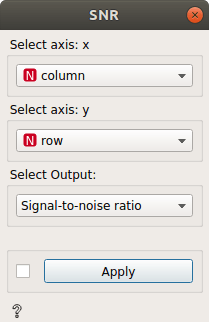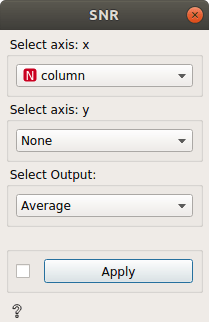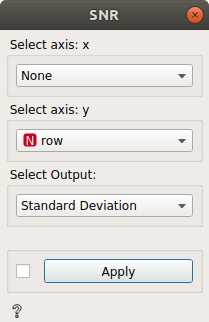# SNR

Signal-to-Noise Ratio (SNR)

Inputs

• Data: input dataset

Outputs

• Signal-to-noise ratio: signal-to-noise ratio dataset
• SNR = $$\frac{\overline{Spectra_{x, y}}}{\sigma _{x, y}}$$
• Averages: averaged dataset
• Averages = $$\overline{Spectra_{x, y}}$$
• Standard Deviation: standard deviation dataset
• Standard Deviation = $$\sigma _{x, y}$$

The SNR widget computes the SNR, average, or standard deviation of spectra. It can output the results of an entire dataset or by coordinates (x, y).

Use Select axis: x to select an axis that will act as the first element for your coordinate system defined by a numeric meta.

Use Select axis: y to select an axis that will act as the second element for your coordinate system defined by a numeric meta.In the example above, the result will be:

output = Signal-to-noise ratio(column, row)

SNR = $$\frac{\overline{Spectra_{column, row}}}{\sigma _{column, row}}$$

If you want to select only one axis:output = Average(x)

Average = $$\overline{Spectra_{column}}$$

oroutput = Standard Deviation(x)

Standard Deviation = $$\sigma _{column}$$

If you want the result of the complete data set, you can just leave both as None.